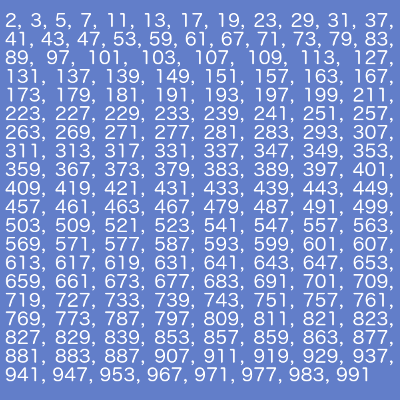# Prime Number Calculator

Share:

Select the type of calculation you want to perform.

Enter the range of numbers, from which you need to identify and list down the prime numbers and press Generate / Check Prime button. NOTE: The difference between the ending number and starting number should not be more than 1000.

The maximum limit is 8,000,000.

Result:

This prime number calculator (Generator & Checker) has four options to find prime number.

1. ### Finding Prime Numbers Between 2 Numbers

If you want to find the prime numbers between 2 given numbers, select the option Primes Between 2 Numbers. This is the default option selected in the drop down box. Enter the range of numbers, from which you need to identify and list down the prime numbers. For example; enter 100 in the Starting Number textbox and 200 in the Ending Number textbox. Once the number range is entered, press Generate / Check Prime button. NOTE: The difference between the ending number and starting number should not be more than 1000. i.e. if the starting number is 258603, then the ending number should be less than or equal to 259603.

2. ### Check For Prime Number

If you want to check whether a number is prime or not, select the option Check For Prime Number. After selecting this option, enter the number you want to check for prime in the textbox and press Generate / Check Prime button.

3. ### Find The Next prime Number

If you want to find the next prime number for the given natural number, select the option Find Next Prime Number. After selecting this option, enter a number in the text box and press Generate / Check Prime to find the next prime of the entered number.

4. ### Find The Previous prime Number

If you want to find the previous prime number for the given natural number, select the option Find Previous Prime Number. After selecting this option, enter a number in the text box and press Generate / Check Prime to find the previous prime of the entered number.

## Prime Numbers Chart

### Generating And Printing The Prime Numbers Chart

1. Enter the Starting Number you desire to start the chart.
2. Click the Generate Chart button.
3. Click the Print button to print the chart you have generated.

Starting Number

 100003 100019 100043 100049 100057 100069 100103 100109 100129 100151 100153 100169 100183 100189 100193 100207 100213 100237 100267 100271 100279 100291 100297 100313 100333
 100343 100357 100361 100363 100379 100391 100393 100403 100411 100417 100447 100459 100469 100483 100493 100501 100511 100517 100519 100523 100537 100547 100549 100559 100591
 100609 100613 100621 100649 100669 100673 100693 100699 100703 100733 100741 100747 100769 100787 100799 100801 100811 100823 100829 100847 100853 100907 100913 100927 100931
 100937 100943 100957 100981 100987 100999 101009 101021 101027 101051 101063 101081 101089 101107 101111 101113 101117 101119 101141 101149 101159 101161 101173 101183 101197

## Prime NumbersPrime numbers are natural numbers which can be divided only by one and itself without a remainder. Here is some of the interesting facts about prime number.

1. 0 and 1 are not prime numbers.
2. None of the even numbers are prime numbers except '2' as they are divisible by 2.
3. Any natural number which ends with 5 is not a prime number except '5' as they are divisible by 5.
4. The largest prime number identified yet (Jan 2016) is 274,207,281-1. This prime was discovered on 17th Sep 2015.
5. There is no such efficient formula to identify a prime number. Only sieve theories are available.
6. To efficiently find primes between smaller numbers you can use Sieve of Eratosthenes theory.
7. Some of the cryptography technologies like RSA uses large prime numbers.

## Reference

0
You may also like...